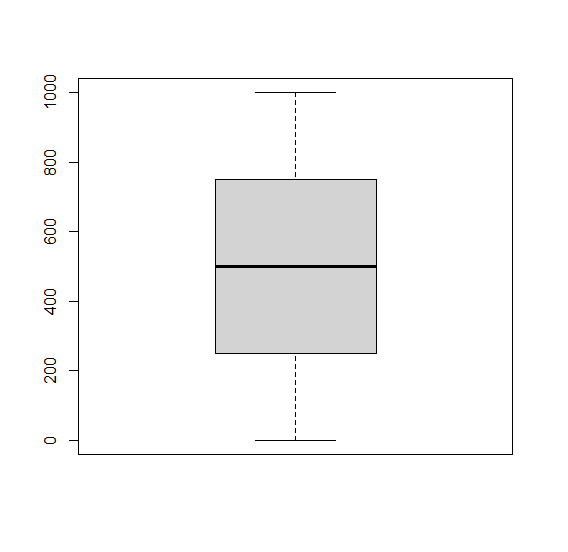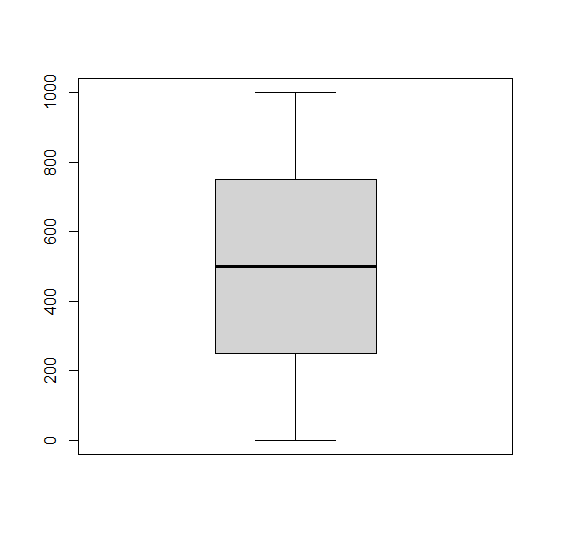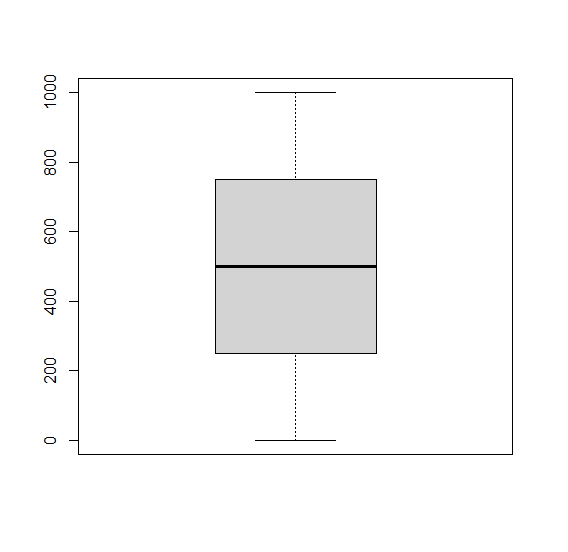# How to change the whisker line type in base R boxplot?

The default boxplot in R has dotted whisker lines and if we want to change it to something else, we can use the whisklty argument.

For Example, if we have a vector called X then we can create the boxplot of X with different whisker line by using the command boxplot(X,whisklty=1). The argument can take different values.

Check out the Examples given below to understand how it works.

## Example

Consider the vector given below −

x<-1:1000
boxplot(x)


## Output

If you execute the above given snippet, it generates the following Output −Add the following code to the above snippet −

x<-1:1000
boxplot(x,whisklty=1)


## Output

If you execute all the above given snippets as a single program, it generates the following Output −Add the following code to the above snippet −

x<-1:1000
boxplot(x,whisklty=9)


## Output

If you execute all the above given snippets as a single program, it generates the following Output −